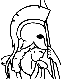#Introduction to Probability, 2nd Edition Table of Contents:

1. Sample Space and Probability

1. Sets
2. Probabilistic Models
3. Conditional Probability
4. Total Probability Theorem and Bayes' Rule
5. Independence
6. Counting
7. Summary and Discussion
8. Problems

2. Discrete Random Variables

1. Basic Concepts
2. Probability Mass Functions
3. Functions of Random Variables
4. Expectation, Mean, and Variance
5. Joint PMFs of Multiple Random Variables
6. Conditioning
7. Independence
8. Summary and Discussion
9. Problems

3. General Random Variables

1. Continuous Random Variables and PDFs
2. Cumulative Distribution Functions
3. Normal Random Variables
4. Joint PDFs of Multiple Random Variables
5. Conditioning
6. The Continuous Bayes' Rule
7. Summary and Discussion
8. Problems

4. Further Topics on Random Variables

1. Derived Distributions
2. Covariance and Correlation
3. Conditional Expectation and Variance Revisited
4. Transforms
5. Sums of Independent Random Variables - Convolution
6. Sum of a Random Number of Independent Random Variables
7. Summary and Discussion
8. Problems

5. Limit Theorems

1. Markov and Chebyshev Inequalities
2. The Weak Law of Large Numbers
3. Convergence in Probability
4. The Central Limit Theorem
5. The Strong Law of Large Numbers
6. Summary and Discussion
7. Problems

6. The Bernoulli and Poisson Processes

1. The Bernoulli Process
2. The Poisson Process
3. Summary and Discussion
4. Problems

7. Markov Chains

1. Discrete-Time Markov Chains
2. Classification of States
4. Absorption Probabilities and Expected Time to Absorption
5. Continuous-Time Markov Chains
6. Summary and Discussion
7. Problems

8. Bayesian Statistical Inference

1. Bayesian Inference and the Posterior Distribution
2. Point Estimation, Hypothesis Testing, and the MAP Rule
3. Bayesian Least Mean Squares Estimation
4. Bayesian Linear Least Mean Squares Estimation
5. Summary and Discussion
6. Problems

9. Classical Statistical Inference

1. Classical Parameter Estimation
2. Linear Regression
3. Binary Hypothesis Testing
4. Significance Testing
5. Summary and Discussion
6. Problems

Index